# Radix Sort in Data Structures

Amit Kumar Ghosh  24 min read
15 Jun 2023
Beginner
371 Views

## What is Radix Sort in Data Structure?

Radix sort is a fascinating algorithm that is widely used in the field of computer science and data analysis. It is a sorting technique that sorts data based on their corresponding digits or numbers. In radix sort, the data are first divided into separate categories based on their least significant digits. These categories are then sorted as well, and the process continues until the most significant digit is reached. Radix sort in data structure is a powerful tool that can efficiently sort data that can be ordered based on numeric or alphabetical values. Despite its complexity, it is one of the fastest sorting algorithms out there and is often preferred over other methods due to its high efficiency and logical approach.

Radix sort is a non-comparative sorting algorithm that sorts integers or strings by processing individual digits or characters. It operates on the principle of "least significant digit (LSD) first," meaning it starts by sorting the numbers based on their least significant digit and progressively moves towards the most significant digit.

Here's a step-by-step overview of how Radix sort works:

1. Initialization: Start by having an unsorted array of integers or strings.
2.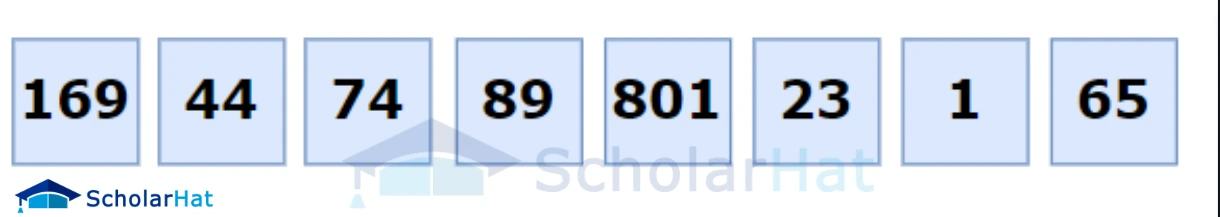3. Find the maximum element: Identify the maximum element in the array to determine the number of digits or characters needed for sorting.
4.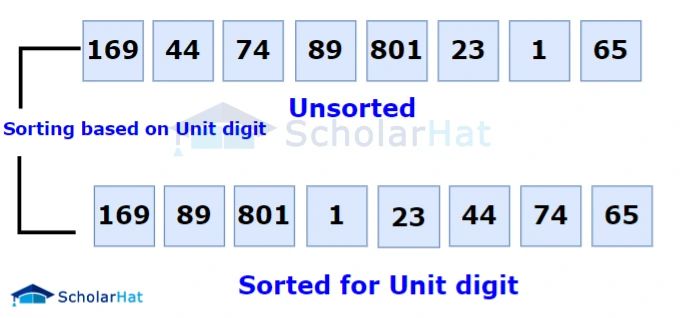5. Perform counting sort for each digit: Iterate through each digit or character position from the least significant digit to the most significant digit.
6.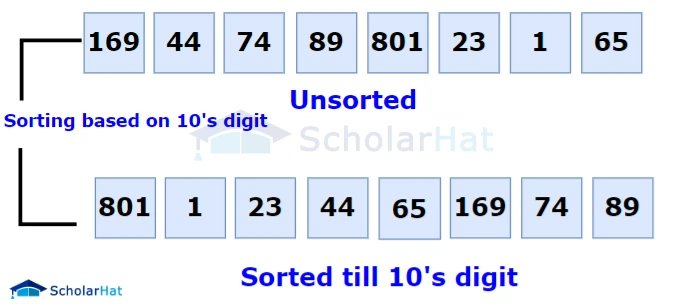• Counting sort: Apply a stable sorting algorithm, such as counting sort, to sort the array based on the current digit or character position. Counting sort is used because it preserves the relative order of elements with the same value.
• Counting occurrences: Count the number of occurrences of each digit or character in the current position. This can be done by iterating through the array and keeping a count for each possible digit or character value.
• Cumulative count: Calculate the cumulative count of the digit or character values. This step helps determine the correct positions of each element in the sorted array.
• Rearrange the elements: Rearrange the elements of the original array based on the current digit or character position, using the counts and cumulative counts obtained in the previous steps. This step ensures that elements with the same digit or character value retain their relative order.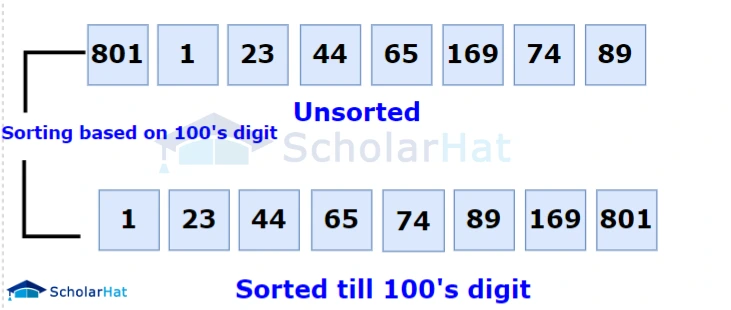7. Repeat the process: Repeat steps 3a to 3d for the next significant digit or character position until all digits or characters have been processed.
8. Final sorted array: After processing all digits or characters, the array will be sorted based on the radix sort algorithm.
9.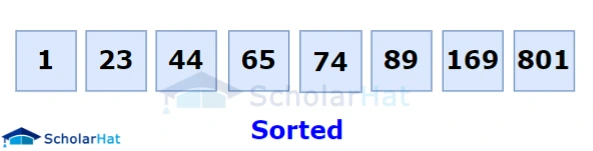Radix sort has a time complexity of O(d * (n + k)), where n is the number of elements, d is the number of digits or characters, and k is the range of possible values for each digit or character. It can be an efficient sorting algorithm when the range of values is not too large compared to the number of elements being sorted.

``````radixSort(array)
d <- maximum number of digits in the largest element
create d buckets of size 0-9
for i <- 0 to d
sort the elements according to ith place digits using countingSort

countingSort(array, d)
max <- find largest element among dth place elements
initialize count array with all zeros
for j <- 0 to size
find the total count of each unique digit in dth place of elements and
store the count at jth index in count array
for i <- 1 to max
find the cumulative sum and store it in count array itself
for j <- size down to 1
restore the elements to array
decrease count of each element restored by 1``````

``````

# Using counting sort to sort the elements in the basis of significant places
def countingSort(array, place):
size = len(array)
output =  * size
count =  * 10

# Calculate count of elements
for i in range(0, size):
index = array[i] // place
count[index % 10] += 1

# Calculate cumulative count
for i in range(1, 10):
count[i] += count[i - 1]

# Place the elements in sorted order
i = size - 1
while i >= 0:
index = array[i] // place
output[count[index % 10] - 1] = array[i]
count[index % 10] -= 1
i -= 1

for i in range(0, size):
array[i] = output[i]

# Main function to implement radix sort
# Get maximum element
max_element = max(array)

# Apply counting sort to sort elements based on place value.
place = 1
while max_element // place > 0:
countingSort(array, place)
place *= 10

data = [121, 432, 564, 23, 1, 45, 788]
print(data)``````
``````
// Radix Sort in Java Programming

import java.util.Arrays;

// Using counting sort to sort the elements in the basis of significant places
void countingSort(int array[], int size, int place) {
int[] output = new int[size + 1];
int max = array;
for (int i = 1; i < size; i++) {
if (array[i] > max)
max = array[i];
}
int[] count = new int[max + 1];

for (int i = 0; i < max; ++i)
count[i] = 0;

// Calculate count of elements
for (int i = 0; i < size; i++)
count[(array[i] / place) % 10]++;

// Calculate cumulative count
for (int i = 1; i < 10; i++)
count[i] += count[i - 1];

// Place the elements in sorted order
for (int i = size - 1; i >= 0; i--) {
output[count[(array[i] / place) % 10] - 1] = array[i];
count[(array[i] / place) % 10]--;
}

for (int i = 0; i < size; i++)
array[i] = output[i];
}

// Function to get the largest element from an array
int getMax(int array[], int n) {
int max = array;
for (int i = 1; i < n; i++)
if (array[i] > max)
max = array[i];
return max;
}

// Main function to implement radix sort
void radixSort(int array[], int size) {
// Get maximum element
int max = getMax(array, size);

// Apply counting sort to sort elements based on place value.
for (int place = 1; max / place > 0; place *= 10)
countingSort(array, size, place);
}

// Driver code
public static void main(String args[]) {
int[] data = { 121, 432, 564, 23, 1, 45, 788 };
int size = data.length;
System.out.println("Sorted Array in Ascending Order: ");
System.out.println(Arrays.toString(data));
}
}``````
``````
// Radix Sort in C++ Programming

#include <iostream>
using namespace std;

// Function to get the largest element from an array
int getMax(int array[], int n) {
int max = array;
for (int i = 1; i < n; i++)
if (array[i] > max)
max = array[i];
return max;
}

// Using counting sort to sort the elements in the basis of significant places
void countingSort(int array[], int size, int place) {
const int max = 10;
int output[size];
int count[max];

for (int i = 0; i < max; ++i)
count[i] = 0;

// Calculate count of elements
for (int i = 0; i < size; i++)
count[(array[i] / place) % 10]++;

// Calculate cumulative count
for (int i = 1; i < max; i++)
count[i] += count[i - 1];

// Place the elements in sorted order
for (int i = size - 1; i >= 0; i--) {
output[count[(array[i] / place) % 10] - 1] = array[i];
count[(array[i] / place) % 10]--;
}

for (int i = 0; i < size; i++)
array[i] = output[i];
}

// Main function to implement radix sort
void radixsort(int array[], int size) {
// Get maximum element
int max = getMax(array, size);

// Apply counting sort to sort elements based on place value.
for (int place = 1; max / place > 0; place *= 10)
countingSort(array, size, place);
}

// Print an array
void printArray(int array[], int size) {
int i;
for (i = 0; i < size; i++)
cout << array[i] << " ";
cout << endl;
}

// Driver code
int main() {
int array[] = {121, 432, 564, 23, 1, 45, 788};
int n = sizeof(array) / sizeof(array);
printArray(array, n);
}
``````

#### Output

``````The output of the given code will be:
1 23 45 121 432 564 788``````

The complexity of radix sort is typically expressed in terms of the number of digits or bits in the input numbers, as well as the number of elements to be sorted. If there are n elements to be sorted, and each element has d digits or bits, then the time complexity of radix sort is O(d * (n + k)), where k is the range of possible values for each digit or bit.

The radix sort algorithm works by sorting the input numbers based on individual digits or bits, from the least significant digit/bit to the most significant one. In each pass, it distributes the numbers into buckets based on the value of the current digit/bit. The number of passes required is determined by the number of digits/bits in the largest number.

The time complexity of each pass is O(n + k), where n is the number of elements and k is the range of possible values for the current digit/bit. The "n" term accounts for iterating over all the elements, while the "k" term accounts for the overhead of distributing the numbers into buckets. Since there are d passes, the overall time complexity is O(d * (n + k)).

The space complexity of radix sort is determined by the auxiliary space used to store the buckets for each digit/bit. In the case of integers, the number of buckets is typically 10 (for decimal digits) or 2 (for binary bits). Therefore, the space complexity is O(n + k) since the size of the buckets is proportional to the number of elements plus the range of values.

It's worth noting that the actual time complexity of radix sort can vary depending on factors such as the implementation details, the specific data set being sorted, and the choice of base for the digits/bits. However, the general complexity analysis described above provides a good understanding of the radix sort's performance characteristics.

 Time Complexity Best O(n+k) Worst O(n+k) Average O(n+k) Space Complexity O(max) Stability Yes

1. Integer Sorting: Radix sort can efficiently sort integers of fixed size. For example, if you have a large collection of 32-bit integers, the radix sort can sort them in linear time by considering each digit's position.
2. String Sorting: Radix sort can be used to sort strings of fixed length or strings with a common maximum length. By considering each character's position, radix sort can order strings based on their character values.
3. IP Address Sorting: Radix sort is often used to sort IP addresses in network applications. IP addresses can be represented as a sequence of numbers separated by periods (e.g., 192.168.0.1). Radix sort can sort IP addresses by considering each octet (digit between periods) from left to right.
4. Phone Number Sorting: In certain applications, you may need to sort phone numbers based on their numerical value. Radix sort can be used to sort phone numbers by considering each digit's position, providing a fast and efficient sorting method.
5. Data Radix Transformation: Radix sort can also be used as a preprocessing step to transform data into a different Radix representation. For example, it can convert numbers from decimal to binary or hexadecimal representation. This transformation can be useful in various algorithms and computations.

• Efficiency for large data sets: Radix sort has a linear time complexity of O(kn), where n is the number of elements to be sorted, and k is the average length of the elements. This makes it efficient for sorting large data sets, especially when the number of elements is significantly larger than the average length of the elements.
• Stable sorting: Radix sort is a stable sorting algorithm, which means that elements with equal keys maintain their relative order after sorting. This property is important when sorting objects with multiple keys or sorting based on multiple criteria.
• Suitable for fixed-length keys: Radix sort is particularly effective when sorting elements with fixed-length keys. It can process each digit or character position independently, making it well-suited for sorting strings, integers, or other data types with a fixed number of digits or characters.
• No dependency on comparison operations: Unlike comparison-based sorting algorithms like quicksort or mergesort, radix sort does not rely on comparison operations between elements. Instead, it leverages the inherent structure of the data being sorted. This can make Radix sort more efficient in certain cases, especially when the cost of comparisons is high.

• Limited Applicability: Radix sort is primarily suitable for sorting fixed-size integers or strings with a consistent length. It becomes less efficient when dealing with variable-length inputs.
• Space Complexity: Radix sort requires additional space to store intermediate results during sorting. The amount of space required depends on the range of values being sorted. In some cases, this can lead to increased memory usage, especially when sorting large datasets.
• Non-Comparison Sort: Radix sort is a non-comparison-based sorting algorithm, which means it does not compare elements directly. While this can be advantageous in terms of performance, it also means that the radix sort does not generalize well to arbitrary data types. It is not suitable for sorting complex objects with multiple attributes or custom-defined ordering.
• Stability: Radix sort is not a stable sorting algorithm. Stability refers to the preservation of the relative order of elements with equal keys. If there are multiple elements with the same key, the original order may not be maintained after sorting, which can be important in certain applications.
• Performance Trade-offs: Although radix sort can be very efficient for sorting integers or strings with a fixed length, its performance can degrade when the number of digits or characters in the input grows significantly. Sorting large numbers or long strings can result in increased runtime, making radix sort less favourable compared to other sorting algorithms for such scenarios.
• Implementation Complexity: Implementing radix sort can be more complex than some other sorting algorithms, especially for beginners or those unfamiliar with the algorithm. The process of partitioning elements based on digits or characters requires careful handling and may involve additional overhead compared to simpler sorting techniques.
##### Summary

In conclusion, Radix sort is an efficient sorting algorithm that can prove invaluable when dealing with large sets of data. Its time complexity of O(nk), where k is the number of bits in each key, makes it stand out from other algorithms since its running time grows at a linear rate. This allows users to quickly and reliably sort through large sets of numbers, which is why it’s employed in many digital sorting applications. Furthermore, Radix Sort also works best when the keys aren’t too big or complicated, which actually makes sense since the more digits or bytes than there are variables per entry, the slower the algorithm will run.

Similar Articles